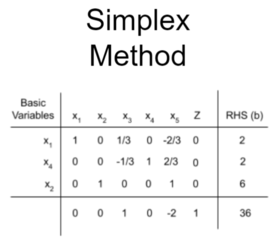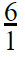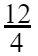Simplex Method of Solving Linear Programming ProblemsSimplex Method

The Simplex method is an approach for determining the optimal value of a linear program by hand.  The method produces an optimal solution to satisfy the given constraints and produce a maximum zeta value.  To use the Simplex method, a given linear programming model needs to be in standard form, where slack variables can then be introduced.  Using the tableau and pivot variables, an optimal solution can be reached.

Slack Variable

Slack variables are additional variables that are introduced into the linear constraints of a linear program to transform them from inequality constraints to equality constraints.

Surplus Variable

Surplus variables are variables subtracted into the linear constraints of a linear program to transform them from inequality constraints to equality constraints.

If the inequality is ≤ (less than or equal), then we add a slack variable + S to change ≤  to =.

For example: 2x1 + x2 ≤ 3 is an inequality.

Then, 2x1 + x2 + s = 3; s is the slack variable

If the inequality is ≥(greater than or equal), then we subtract a surplus variable - S to change ≥ to =.

For example: 2x1 + 3x2 ≥ 5 is an inequality.

Then, 2x1 + 3x2 - s = 5; s is the surplus variable

Standard Form of a maximization problem in two variables

Standard form is the baseline format for all linear programs before solving for the optimal solution and has three requirements: (1) must be a maximization problem, (2) all linear constraints must be in a less-than-or-equal-to inequality, (3) all variables are non-negative.

Example:

Z = 7x1 + 5x2

subject to

x1 + 2x2 ≤ 6

4x1 + 3x2 ≤ 12

x1, x2 ≥ 0

Basic Solution

Given a system of m linear equations with n variables (m < n). Any solution which is obtained by solving for m variables keeping the remaining (n–m) variables zero is called a basic solution.

Basic feasible Solution

A basic solution, which also satisfies the non-negative constraints, is called a basic feasible solution.

Bounded, Unbounded, Empty Solutions

If the value of objective function Z has both a maximum value and minimum value, such a solution is a bounded solution.

If the value of the objective function Z can be increased or decreased indefinitely, such solutions are called unbounded solutions. An unbounded solution has minimum values but no maximum value.

An empty solution will have no maximum or minimum value.

Fundamental Theorem of LP

The fundamental theorem of linear programming says that if there is a solution, it occurs on the boundary of the feasible region, not inside the region.

Basic Variables

Basic variables are variables that are non-negative in terms of the optimal solution.

Non-Basic Variables

Non-basic variables are variables that are zero in terms of the optimal solution.

Simplex Tableau

Simplex tableau is used to perform row operations on the linear programming model as well as for checking optimality.

Optimality Check

Optimal solutions of a maximization linear programming model are the values assigned to the variables in the objective function to give the largest zeta value. The optimal solution would exist on the corner points of the graph of the entire model.

Exercise 1 (Step-wise explanation)

Use the simplex method to find the optimal solutions of the following LP Problem.

Max. Z = 7x1 + 5x2

subject to

x1 + 2x2 ≤ 6

4x1 + 3x2 ≤ 12

x1, x2 ≥ 0

Solution:

Step 1: Standard form

Standard form is necessary because it creates an ideal starting point for solving the Simplex method as efficiently as possible.

Max. P = 7x1 + 5x2

subject to

x1 + 2x2 ≤ 6

4x1 + 3x2 ≤ 12

x1, x2 ≥ 0

Note:

To transform a minimization linear program model into a maximization linear program model, simply multiply both the left and the right sides of the objective function by -1.

-1 × [-Z = -8x1 - 10x2 - 7x3]

Z = 8x1 + 10x2 + 7x3

Maximize: Z = 8x1 + 10x2 + 7x3

Transforming linear constraints from a greater-than-or-equal-to inequality to a less-than-or-equal-to inequality can be done similarly as what was done to the objective function.  By multiplying by -1 on both sides, the inequality can be changed to less-than-or-equal-to.
-1 × [x1 - 5x2 - x3 ≥ -8 ]
x1 + 5x2 +  x3 ≤ 8

Step 2: Determine Slack Variables

Let x3 and x4 be non-negative slack variables,

x1 + 2x2 + x3 = 6

4x1 + 3x2 + x4 = 12

7x1 + 5x2 = P

Now, the given LP problem in its standard form is,

1.x1 + 2.x2 + 1.x3 + 0.x4 + 0.P = 6

4.x1 + 3.x2 + 0.x3 + 1.x4 + 0.P = 12

-7.x1 + -5.x2 + 0.x3 + 0.x4 + 1.P = 0

Step 3: Setting up the Tableau

The tableau consists of the coefficient corresponding to the linear constraint variables and the coefficients of the objective function.

The equations in initial simplex tableau is as follows:Step 4: Check Optimality

To check optimality using the tableau, all values in the last row must contain values greater than or equal to zero. If a value is less than zero, it means that variable has not reached its optimal value.  As seen in the previous tableau, two negative values exist in the bottom row indicating that this solution is not optimal. If a tableau is not optimal, the next step is to identify the pivot element to base a new tableau on.

Step 5: Identify Pivot Element
The pivot element can be identified by looking at the bottom row of the tableau and the indicator. Pick the smallest negative value in the bottom row. That column containing the smallest negative value would be the pivot column. One of the values lying in the pivot column will be the pivot element. To find the indicator, divide the beta values of the linear constraints by their corresponding values from the pivot column.∵ -7 is the most -ve value(smallest value), so, the first column is the pivot column.= 6 and= 3(min) [3<6]

∴ 4 is the pivot element.

Step 6: Create the New Tableau

1) To optimize the pivot variable, it will need to be transformed into a unit value (value of 1). To transform the value, multiply the row containing the pivot variable by the reciprocal of the pivot value. In the example below, the pivot variable is originally 4, so multiply the entire row by 1/4.

R2 → R2/42) After the unit value has been determined, the other values in the column containing the unit value will become zero.  This is because the x2 in the second constraint is being optimized, which requires x2 in the other equations to be zero.

R1 → R1 - R2R3 → 7R2 + R3Once the new tableau has been completed, the model can be checked for an optimal solution.

∴ All the entries in the last row are non-negative.

So, the optimal solution is obtained.

So, maximum P=21 when x1= 3 and x2= 0

Finally, Max P = 7x1 + 5x2 = 7.3 + 0 = 21

Step 8: Identify New Pivot Variable

If the solution has been identified as not optimal, a new pivot element will need to be determined.  Steps are repeated from Step 5 and optimality is checked until optimal values can be obtained.

Exercise 2

Use the simplex method to find the optimal solutions of the following LP Problem.

Max. Z = 3x1 + 5x2

subject to

3x1 + 2x2 ≤ 18

x1 ≤ 4

x2 ≤ 6

x1, x2 ≥ 0

Solution:

Let x3, x4 and x5 be non-negative slack variables,

3x1 + 2x2 + x3 = 18

x1+ x4 = 4

x2 + x5 = 6

3x1 + 5x2 = Z

Now, the given LP problem in its standard form is,

3.x1 + 2.x2 + 1.x3 + 0.x4 + 0.x5 +  0.Z = 18

1.x1 + 0.x2 + 0.x3 + 1.x4 + 0.x5 +  0.Z = 4

0.x1 + 1.x2 + 0.x3 + 0.x4 + 1.x5 +  0.Z = 6

3.x1 + 5.x2 + 1.x3 + 0.x4 + 0.x5 +  0.Z = 0

The equations in initial simplex tableau is as follows:∵ -5 is the most -ve value(smallest value), so, the second column is the pivot column.= 9 and= 5(min) [5<18]

∴ 1 is the pivot element.

R1 → R1 - 2R3

R4 → R4 + 5R3∵ -3 is the most -ve value(smallest value), so, the first column is the pivot column.= 2(min)  and= 4 [2<4]
∴ 3 is the pivot element.

R1 → R1/3R2 → R2 - R1

R4 → R4 + 3R1∴ All the entries in the last row are non-negative.

So, the optimal solution is obtained.

So, maximum Z=36 when x1= 2 and x2= 6

Finally, Max Z= 3x1+ 5x2 = 3.2 + 5.6 = 36

Dual of LP Problem

If the LP problem is of maximization type then it can be solved by simplex method.

But if the given LP Problem is of the minimization type then it can be solved after changing itself into the maximization problem, this is known as the dual problem of the given LP Problem.

Dual of a Minimization LP Problem

Min. C= ax + by

subject to

a1x + b1y ≤ c1

a2x + b2y ≤ c2

c1, c2 ≥ 0

x, y ≥ 0

Here,

A= Augmented matrix formed from the constraints and the objective functionThe corresponding dual problem of the given minimization problem is as follows:

Max.  P = c1x + c2y

subject to

a1u + b1v ≤ a

a2u + b2v ≤ b

c1, c2 ≥ 0

u, v ≥ 0B is the transposed matrix of A i.e. B = AT

By duality theorem, Minimize C = Maximize P

Exercise 3

Minimize  P = 7x1 + x2

subject to

3x1 -  2x2 ≤ -6

x1+ 3x2 ≥ 15

x1, x2 ≥ 0

Solution:

Standard Form:

Minimize  P = 7x1 + x2

subject to

3x1 -  2x2 ≤ -6 or,-3x1 + 2x2 ≥ 6

x1+ 3x2 ≥ 15

x1, x2 ≥ 0

Let A be the augmented matrix formed by the coefficients of constraints with objective function at the bottom.Now, corresponding dual LP is,

Max P* = 6y1 + 15y2

subject to

-3y1 + y2 ≤ 7

2y1+ 3y2 ≤ 1

y1, y2 ≥ 0

Let x1 and x2 be non-negative slack variables,

-3y1 + y2 + x1 = 7

2y1+ 3y2 + x2 = 1

-6y1 + -15y2 + P* = 0

Now, the given LP problem in its standard form is,

-3.y1 + 1.y2 + 1.x1 + 0.x2 + 0.P* = 7

2.y1+ 3.y2  + 0.x1 + 1.x2 + 0.P* = 1

-6y1 + -15y2+ 0.x1 + 0.x2 + 1.P* = 0

The equations in initial simplex tableau is as follows:∵ -15 is the most -ve value(smallest value), so, the second column is the pivot column.= 7 and= 0.33 (min) [0.33<7]

∴ 3 is the pivot element.

R2 → R2/3R1 → R1 - R2

R3 → R3 + 15R2∴ All the entries in the last row are non-negative.

So, the optimal solution is obtained.

So, maximum P*=5 when x1= 0 and x2= 5

Finally, Max P*= 7x1 + x2 = 0 + 5 = 5.